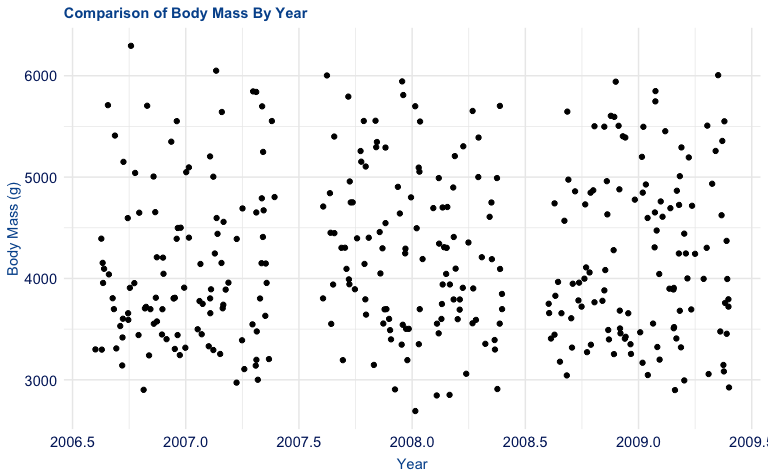# theme_duke()

This vignette is intended to demonstrate the breadth of theme_duke() and its various applications. Similar to the other functions within the theme(), this function can be applied to all visualizations made with ggplot2.

# Plot Examples

For these visualizations, we will use the penguins dataset from the palmerpenguins package.

library(palmerpenguins)
library(ggplot2)
library(duke)

## Scatter Plot

p <- ggplot(penguins, aes(bill_length_mm, flipper_length_mm)) +
geom_point(aes(colour = species)) +
labs(
title = "Bill Length vs. Flipper Length",
caption = "There are three different species of penguins.",
x = "Bill Length (mm)",
y = "Flipper Length (mm)"
)

p +
theme_duke()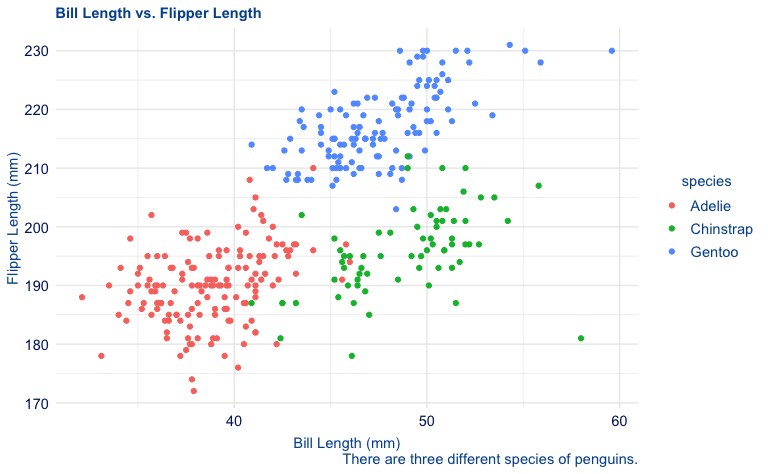## Bar Plot

p <- ggplot(penguins, aes(species)) +
geom_bar(aes(fill = species)) +
labs(
title = "Species Count",
caption = "There are three different species of penguins.",
x = "Species",
y = "Count"
)

p +
theme_duke()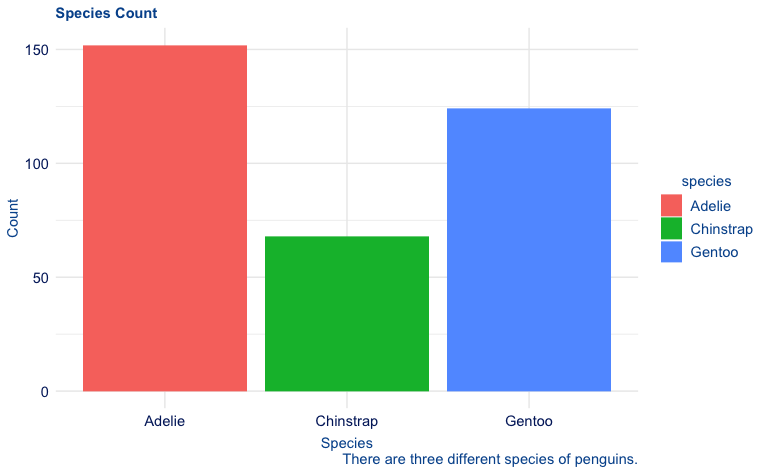## Histogram

p <- ggplot(penguins, aes(body_mass_g)) +
geom_histogram(aes(fill = species)) +
labs(
title = "Distribution of Penguin Body Mass",
caption = "There are three different species of penguins.",
x = "Body Mass (g)",
y = "Count"
)

p +
theme_duke()
#> stat_bin() using bins = 30. Pick better value with binwidth.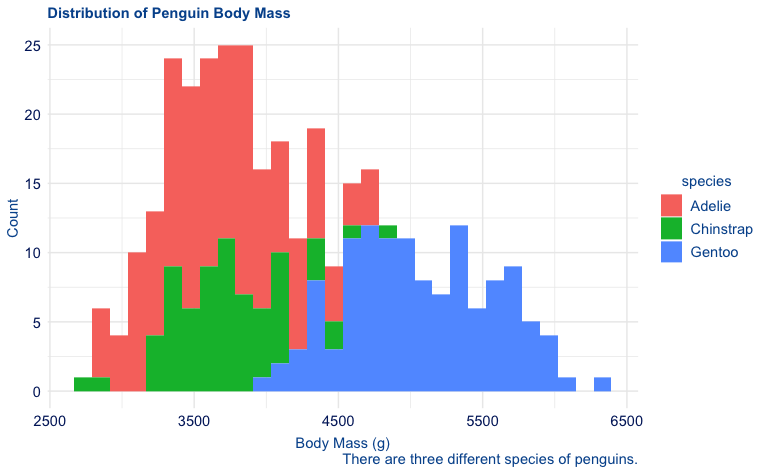## Box Plot

p <- ggplot(penguins, aes(sex, body_mass_g)) +
geom_boxplot() +
labs(
title = "Comparison of Body Mass By Sex",
x = "Sex",
y = "Body Mass (g)"
)

p +
theme_duke()
#> Warning: Removed 2 rows containing non-finite values (stat_boxplot()).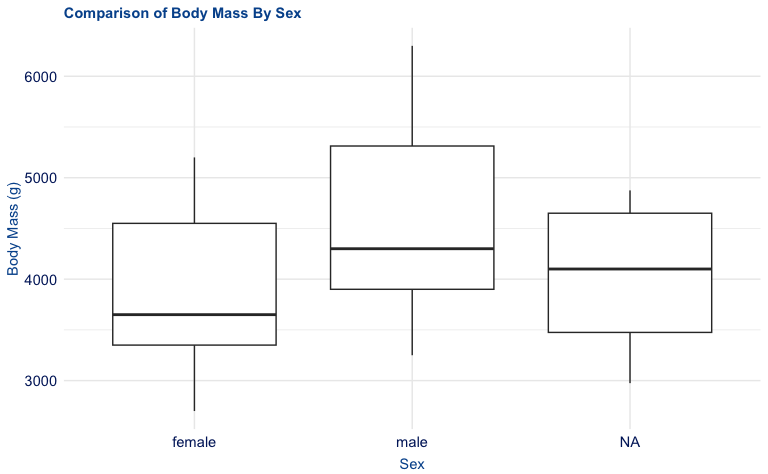## Density Plot

p <- ggplot(penguins, aes(bill_depth_mm)) +
geom_density() +
labs(
title = "Density of Penguin Bill Depth",
x = "Bill Depth (mm)",
y = "Densiy"
)

p +
theme_duke()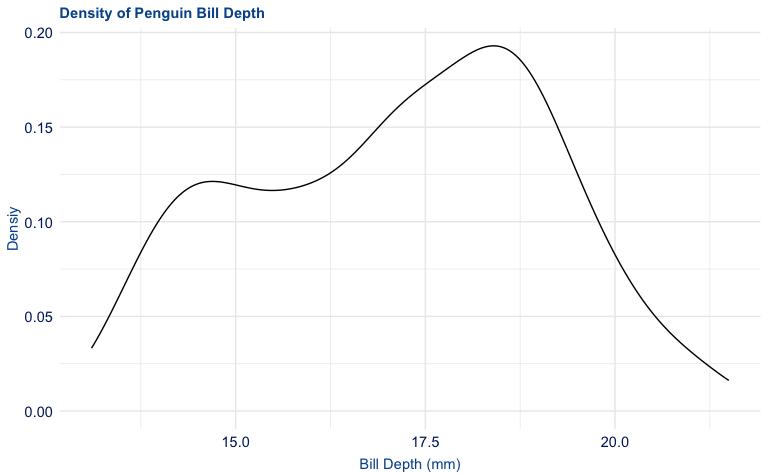## Jitter Plot

p <- ggplot(penguins, aes(year, body_mass_g)) +
geom_jitter() +
labs(
title = "Comparison of Body Mass By Year",
x = "Year",
y = "Body Mass (g)"
)

p +
theme_duke()